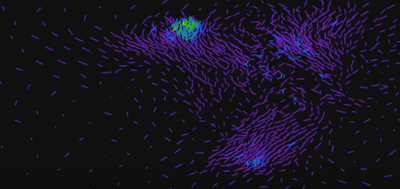Choose Language Hide Translation Bar
Video flock/swarm/school

Spray Paintwas a poor attempt at flocking behavior.  I think the part I was missing was alignment, which http://gamedevelopment.tutsplus.com/tutorials/the-three-simple-rules-of-flocking-behaviors-alignment... steered me toward.  Here's a new video.And here's the JSL for creating the bitmaps (again, no apologies...you'll need to remove the neighborhood and nnear calculation from the iterate function if you want to play with the sliders.  This is the final JSL that ran for several hours to make all the bitmaps, not the early JSL when I was trying to find some parameter values that would make interesting results.)

// ideas borrowed from

neighborhood = 2; // radius of near-by neighborhood

nnear=20; // number of neighbors to find in the neighborhood

a=.04;b=0.08;c=0.2;

w=.8;//1-w is pull to center

speed=8;//smaller is faster

x = 1;

y = 2;

dx = 3;

dy = 4;

color=5;

nvar = 5;

nflock = 1700;

flock = J( nflock, nvar, . );

// set initial positions from 10 to 90 and initial velocities less than 1

Parallel Assign(

{x = x, y = y, dx = dx, dy = dy, nflock = nflock,color=color},

flock[i, v] = If( v < dx,

100*(i+100)/(nflock+200),//Random Integer( 10, 90 ),

if(v<color,Max( -1, Min( 1, /*Random Normal( 0, 1 )*/(i-nflock/2)/(nflock/2) ) ), rgbcolor(0,0,0))

)

);

frame = 0;

iterate = Function( {},

{temp = flock, iflock, irow, kdt = KDTable( flock[0, 1 :: 2] ), dxsum, dysum, xsum, ysum, myx, myy, xdif, ydif, neighbor, nneighbors, len},

nnear = 10*(1.1+sin(frame/2000)); // one to twenty nearest neighbors

neighborhood = 1*(1+cos(frame/3000)); // zero to two neighborhood

{rows, dist} = kdt << KNearestRows( {nnear, neighborhood} );

frame++;

For( iflock = 1, iflock <= nflock, iflock++,

myx = temp[iflock, x];

myy = temp[iflock, y];

dxsum = 0;

dysum = 0; // sum of neighbor velocities

xsum = 0;

ysum = 0; // sum of neighbor positions

xdif = 0;

ydif = 0; // sum of neighbor distances

nneighbors = 0;

For( irow = 1, irow <= nnear & (neighbor = rows[iflock, irow]) != 0, irow++,

nneighbors++;

dxsum += temp[neighbor, dx];

dysum += temp[neighbor, dy];

if(1,

xsum += temp[neighbor, x];

ysum += temp[neighbor, y];

if(1,

xdif += (myx - temp[neighbor, x]); // vector away from neighbor

ydif += (myy - temp[neighbor, y]);

))

);

len = Sqrt( dxsum * dxsum + dysum * dysum );

If( len != 0,

dxsum /= len; // this makes the velocity become 1, in the group's direction

dysum /= len;

,

dxsum = 0;

dysum = 0;

);

If( nneighbors,

xc = 50+40*sin((choose(1+mod(iflock,3),3,5,7)*frame)/2207);

yc = 50+40*cos((choose(1+mod(iflock,3),11,13,17)*frame)/3067);

xsum = ((1-w)*xc+(w*(xsum) / (nneighbors))) - myx; // this vector provides direction to the group center

ysum = ((1-w)*yc+(w*(ysum) / (nneighbors))) - myy;

len = Sqrt( xsum * xsum + ysum * ysum );

if(len!=0,xsum/=len;ysum/=len,xsum=0;ysum=0);// normalized to 1

len = Sqrt( xdif * xdif + ydif * ydif );

if(len!=0,xdif/=len;ydif/=len,xdif=0;ydif=0); // normalized to 1

,

xsum = 0;

ysum = 0;

xdif = 0;

ydif = 0;

);

flock[iflock, dx] += a*dxsum + b*xsum + c*xdif;

if( abs(flock[iflock, dx])>1, flock[iflock, dx]*=.9);

flock[iflock, x] += flock[iflock, dx]/speed;

if((flock[iflock, x] < -5 & flock[iflock, dx] < 0)|(flock[iflock, x] >105 & flock[iflock, dx] > 0), flock[iflock, dx] = - flock[iflock, dx]);

flock[iflock, dy] += a*dysum + b*ysum + c*ydif;

if( abs(flock[iflock, dy])>1, flock[iflock, dy]*=.9);

flock[iflock, y] += flock[iflock, dy]/speed;

if((flock[iflock, y] < -5 & flock[iflock, dy] < 0)|(flock[iflock, y] >105 & flock[iflock, dy] > 0), flock[iflock, dy] = - flock[iflock, dy]);

flock[iflock,color]=heatcolor((nneighbors)/(nnear),"spectral");//heatcolor((nneighbors-1)/(nnear+10),"jet");//3+nneighbors;//hlscolor(.4-.6*(nneighbors)/(nnear),.5,1);//

);

);

iterate();

New Window( "Flock",

V List Box(

gb = Graph Box(

suppressaxes,

framesize( 1922, 1082 ),

<<backgroundcolor( RGB Color( 0, 0, 0 ) ),

Pen Size( 3 );

For( i = 1, i <= nflock, i++,

Pen Color( flock[i, color] );

Line(  {flock[i, x] - flock[i, dx], flock[i, y] - flock[i, dy]}, {flock[i, x], flock[i, y]} );

);

),

H List Box(

Text Box( "    Alignment" ),

Slider Box( 0, .16, a ),

Text Box( "    Cohesion" ),

Slider Box( 0, .2, b ),

Text Box( "  Separation" ),

Slider Box( 0, .4, c ),

Text Box( "    Neighborhood" ),

Slider Box( 0, 30, neighborhood ),

,

Text Box( "    NNear" ),

Slider Box( 1, 30, nnear ),

Text Box( "    Speed" ),

Slider Box( .5, 30, speed )

)

)

);

While( frame<=27000,

gb << inval;

gb << updatewindow;

Wait( 0 );

temp=gb[framebox(1)]<<getpicture;

pixels=temp<<getpixels;

pixels=pixels[3::1082,3::1922];

temp<<setpixels(pixels);

temp<<saveimage("\$temp/f"||right(char(frame,9),9,"0")||".png");

iterate();

);

Article Labels

There are no labels assigned to this post.

Article Tags